Appendix C - The ZX81 for those that understand BASIC

General

If you already know BASIC then you should not have much trouble using the ZX81; but it has one or two idiosyncrasies.

(i) Words are not spelled out, but have keys of their own - this is dealt with in chapter 2 (for keywords & shifted keys) & chapter 5 (for function names). In the text, these words are printed in BOLD TYPE.

(ii) ZX81 BASIC lacks READ, DATA & RESTORE (but see exercise 3 of chapter 22 concerning this), user-defined functions (FN & DEF; but VAL can sometimes be used), & multi-statement lines.

(iii) The string handling facilities are comprehensive but non-standard - see chapter 21, & also chapter 22 (for string arrays).

(iv) The ZX81 character set is completely its own.

(v) The television display is not in general memory-mapped.

(vi) If you are accustomed to using PEEK & POKE on a different machine, remember that all the addresses will be different on the ZX81.

Speed

The machine works at two speeds, called compute & display mode, & fast mode.

In compute & display, the television picture is generated continuously & computing is done during the blank parts at the top & bottom.

In fast mode, the television picture is turned off during computing, & is only displayed at the end of the program, while waiting for INPUT data, or during a pause (see PAUSE).

Fast mode runs about four times as fast, & should be used for programs with a lot of computing as opposed to output, or when typing in long programs.

Switching between speeds is done with the FAST & SLOW statements (q.v.).

The keyboard

ZX81 characters comprise not only the single symbols (letters, digits, etc.), but also the compound tokens (keywords, function names, etc.; these are printed here in BOLD TYPE) & all these are entered from the keyboard rather than being spelled out. To fit this in, some keys have up to five distinct meanings, given partly by shifting the keys (i.e. pressing the SHIFT key at the same time as the required one) & partly by having the machine in different modes.

The mode is indicated by the cursor, an inverse video letter that shows where the next character from the keyboard will be inserted.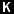mode (for keywords) occurs automatically when the machine is expecting a command or program line (rather than INPUT data), & from its position on the line it knows it should expect a line number or a keyword. This is at the beginning of the line, or just after some digits at the beginning of the line, or just after THEN. If unshifted, the next key will be interpreted as either a keyword (these are mostly written above the keys), or a digit.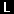mode (for letters) normally occurs at all other times. If unshifted, the next key will be interpreted as the main symbol on that key.

In bothmodes, shifted keys will be interpreted as the subsidiary red character in the top right-hand corner of the key.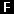mode (for functions) occurs after FUNCTION (shifted NEWLINE) is pressed, & lasts for one key depression only. That key will be interpreted as a function name, these appearing under the keys.mode for graphics occurs after GRAPHICS (shifted 9) is pressed, and lasts until it is pressed again. An unshifted key will give the inverse video of itsmode interpretation; a shifted key will as well, provided that it is a symbol, but if the shifted key would normally give a token, in graphics mode it gives the graphics symbol that appears in the bottom right hand corner of the key.

The screen

This has 24 lines, each 32 characters long, and is divided into two parts. The top part is at most 22 lines, and displays either a listing or program output. The bottom part, at least two lines, is used for inputting commands, program lines and INPUT data, and also for displaying reports.

Keyboard input: this appears in the bottom half of the screen as it is typed, each character (single symbol or compound token) being inserted just before the cursor. The cursor can be moved left with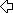(shifted 5) or right with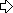(shifted 8). The character before the cursor can be deleted with RUBOUT (shifted 0). (Note:- the whole line can be deleted by typing EDIT (shifted 1) followed by NEWLINE; or, if it is INPUT data, just by typing EDIT.)

When NEWLINE is pressed, the line is executed, entered into the program, or used as INPUT data as appropriate, unless it contains a syntax error. In this case the symbolappears just before the error.

As program lines are entered, a listing is displayed in the top half of the screen. The manner in which the listing is produced is rather complicated, and explained more fully in chapter 9, exercise 6. The last line to be entered is called the current line and indicated by the symbol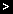, but this can be changed using the keys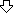(shifted 6) and(shifted 7). If EDIT (shifted 1) is pressed, the current line is brought down to the bottom part of the screen and can be edited.

When a command is executed or a program run, the screen is first of all cleared, & then output is displayed in the top half of the screen and remains until a program line is entered, or NEWLINE is pressed with an empty line, ororis pressed. In the bottom part appears a report of the form m/n where m is a code showing what happened (see appendix B), & n is the number of the last line executed - or 0 for a command. The report remains until a key is pressed (and indicatesmode).

In certain circumstances, the SPACE key acts as a BREAK, stopping the computer with report D. This is recognized

(i) at the end of a statement while a program is running,

(ii) while the computer is looking for a program on tape,

or    (iii) while the computer is using the printer (or by accident trying to use it when it is not there).

The BASIC

Numbers are stored to an accuracy of 9 or 10 digits. The largest number you can get is about 1038, & the smallest (positive) number is about 4 * 10-39.

A number is stored in the ZX81 in floating point binary with one exponent byte e (1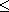255), & four mantissa bytes m (½1). This represents the number m * 2e-128.

Since ½1, the most significant bit of the mantissa m is always 1. Therefore in actual fact we can replace it with a bit to show the sign - 0 for positive numbers, 1 for negative.

Zero has a special representation in which all 5 bytes are 0.

Numeric variables have names of arbitrary length, starting with a letter and continuing with letters and digits. All these are significant, so that for instance LONGNAME & LONGNAMETOO are distinct names. Spaces are ignored.

Control variables for FOR-NEXT loops have names a single letter long.

Numeric arrays have names a single letter long, which may be the same as the name of a simple variable. They may have arbitrarily many dimensions of arbitrary size. Subscripts start at 1.

Strings are completely flexible in length. The name of a string consists of a single letter followed by \$.

String arrays can have arbitrarily many dimensions of arbitrary size. The name is a single letter followed by \$ and may not be the same as the name of a string. All the strings in a given array have the same fixed length, which is specified as an extra, final dimension in the DIM statement. Subscripts start at 1.

Slicing: Substrings of strings may be specified using slicers.

A slicer can be

(i) empty

or

(ii) numerical expression

or

(iii) optional numerical expression TO optional numerical expression

& is used in expressing a substring either by

(a) string expression (slicer)

or by

(b) string array variable (subscript,...,subscript, slicer)

which means the same as

string array variable (subscript,...,subscript)(slicer)

In (a), suppose the string expression has the value s\$.

If the slicer is empty, then the result is s\$ considered as a substring of itself.

If the slicer is a numerical expression with value m, then the result is the mth character of s\$ (a substring of length 1).

If the slicer has the form (iii), then suppose the first numerical expression has the value m (the default value is 1), & the second, n (the default value is the length of s\$).

If 1the length of s\$ then the result is the substring of s\$ starting with the mth character & ending with the nth.

If 0m then the result is the empty string.

Otherwise, error 3 results.

Slicing is performed before functions or operations are evaluated, unless brackets dictate otherwise.

Substrings can be assigned to (see LET).

The argument of a function does not need brackets if it is a constant or a (possibly subscripted or sliced) variable.

 Function Type of operand (x) Result ABS number Absolute magnitude. ACS number Arccosine in radians. Error A if x not in the range -1 to +1. AND binary operation, right operand always a number Numeric left operand: A AND B = A if B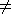0, 0 if B = 0 String left operand: A\$ AND B = A\$ if B0, "" if B = 0 ASN number Arcsine in radians. Error A if x not in the range -1 to +1. ATN number Arctangent in radians. CHR\$ number The character whose code is x, rounded to the nearest integer. Error B if x not in the range 0 to 255. CODE string The code of the first character in x (or 0 if x is the empty string). COS number (in radians) Cosine EXP number ex INKEY\$ none Reads the keyboard. The result is the character representing (inmode) the key pressed if there is exactly one, else the empty string. INT number Integer part (always rounds down). LEN string Length LN number Natural logarithm (to base e) Error A if x0 NOT number 0 if x0, 1 if x = 0. NOT has priority 4. OR binary operation, both operands numbers A OR B = 1 if B0, A if B = 0 OR has priority 2. PEEK number The value of the byte in memory whose address is x (rounded to the nearest integer). Error B if x not in the range 0 to 65535. PI none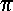(3.14159265..) RND none The next pseudo-random number y in a sequence generated by taking the powers of 75 modulo 65537, subtracting 1 & dividing by 65536. 0y1. SGN number Signum: the sign (-1, 0 or +1) of x. SIN number (in radians) Sine SQR number Square root Error B if x < 0 STR\$ number The string of characters that would be displayed if x were printed. TAN number (in radians) Tangent USR number Calls the machine code subroutine whose starting address is x (rounded to the nearest integer). On return, the result is the contents of the bc register pair. Error B if x is not in the range 0 to 65535. VAL string Evaluates x (without its bounding quotes) as a numerical expression. Error C if x contains a syntax error, or gives a string value. Other errors possible, depending on the expression. - number Negation

The following are binary operations:
 + Additional (on numbers), or concatenation (on strings). - Subtraction * Multiplication / Division ** Raising to a power. Error B if the left operand is negative. = Equals* > Greater than* < Less than* <= Less than or equal to* >= Greater than or equal to* <> Not equal to*
* Both operands must be of the same type. The result is a number, 1 if the comparison holds & 0 if it does not.

Functions & operations have the following priorities:
 Operation Priority Subscripting & slicing 12 All functions except NOT and unary minus 11 ** 10 Unary minus 9 * , / 8 + , - (binary -) 6 = , > , < , <= , >=, <> 5 NOT 4 AND 3 OR 2

Statements

In this list,
 α represents a single letter v represents a variable x,y,z represent numerical expressions m,n represent numerical expressions that are rounded to the nearest integer e represents an expression f represents a string valued expression s represents a statement

Note that arbitrary expressions are allowed everywhere (except for the line numbers at the beginning of a statement).

All statements except INPUT can be used either as commands or in programs (although they may be more sensible in one than the other).

 CLEAR Deletes all variables, freeing the space they occupied. CLS (Clear Screen) Clears the display file. See chapter 27 concerning the display file. CONT Suppose p/q was the last report with a non-zero. Then CONT has the effect GOTO q if p9, GOTO q+1 if p = 9 (STOP statement) COPY Sends a copy of the display to the printer, if attached; otherwise does nothing. Unlike all other commands, a COPY command does not clear the screen first. There must be no spaces before COPY. Report D if BREAK pressed. DIM α(n1,...,nk) Deletes any array with the name α, & sets up an array α of numbers with k dimensions n1,...,nk. Initializes all the values to 0. Error 4 occurs if there is no room to fit the array in. An array is undefined until it is dimensioned in a DIM statement. DIM α\$(n1,...,nk) Deletes any array or string with the name α\$, & sets up an array α\$ of characters with k dimensions n1,...,nk. Initializes all the values to "". This can be considered as an array of strings of fixed length nk, with k-1 dimensions n1,...,nk-1. Error 4 occurs if there is no room to fit the array in. An array is undefined until it is dimensioned in a DIM statement. FAST Starts fast mode, in which the display file is displayed only at the end of the program, while INPUT data is being typed in, or during a pause. FOR α=x TO y FOR α=x TO y STEP 1 FOR α=x TO y STEP z Deletes any simple variable α, & sets up a control variable with value x, limit y, step z, & looping address 1 more than the line number of the FOR statement (-1 if it is a command). Checks if the initial value is greater (if step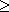0) or less (if step < 0) than the limit, & if so then skips to statement NEXT α at the beginning of a line. See NEXT α. Error 4 occurs if there is no room for the control variable. GOSUB n Pushes the line number of the GOSUB statement onto a stack; then as GOTO n Error 4 can occur if there are not enough RETURNs. GOTO n Jumps to line n (or, if there is none, the first line after that). IF x THEN s If x is true (non-zero) then s is executed. The form 'IF x THEN line number' is not allowed. INPUT v Stops (with no special prompt) & waits for the user to type in an expression; the value of this is assigned to v. In fast mode, the display file is displayed. INPUT cannot be used as a command; error 8 occurs if you try. If the first character in the INPUT line is STOP, the program stops with report D. LET v=e Assigns the value of e to the variable v. LET cannot be omitted. A simple variable is undefined until it is assigned to in a LET or INPUT statement. If v is a subscripted string variable, or a sliced string variable (substring), then the assignment is Procrustean: the string value of e is either truncated or filled out with spaces on the right, to make it the same length as the variable v. LIST LIST 0 LIST n Lists the program to the television, starting at line n, & makes n the current line. Error 4 or 5 if the listing is too long to fit on the screen; CONT will do exactly the same again. LLIST LLIST 0 LLIST n Like LIST, but using the printer instead of the television. Should do nothing if the printer is not attached. Stops with report D if BREAK pressed. LOAD f Looks for a program called f on tape, & loads it & its variables. If f = "", then loads the first program available. If BREAK is pressed or a tape error is detected, then     (i) if no program has yet been read from tape, stops with report D & old program;     (ii) if part of a program has been read in, then executes NEW. LPRINT ... Like PRINT, but using the printer instead of the television. A line of text is sent to the printer.     (i) when printing spills over from one line to the next,     (ii) after an LPRINT statement that does not end in a comma or a semicolon,     (iii) when a comma or TAB item requires a new line, or     (iv) at the end of the program, if there is anything left unprinted. In an AT item, only the column number has any effect; the line number is ignored (except that the same error conditions arise as for PRINT if it is out of range). An AT item never sends a line of text to the printer. There should be no effect if the printer is absent. Stops with report D if BREAK is pressed. NEW Starts the BASIC system off anew, deleting program & variables, & using the memory up to but not including the byte whose address is in the system variable RAMTOP (bytes 16388 & 16389). NEXT α (i) Finds the control variable α.     (ii) Adds its step to its value.     (iii) If the step0 & the value > the limit; or if the step < 0 & the value < the limit, then jumps to the looping line. Error 1 if there is a simple variable α. Error 2 if there is no simple or control variable α. PAUSE n Stops computing & displays the display file for n frames (at 50 frames per second) or until a key is pressed. 0n65535, else error B. If n32767 then the pause is not timed, but lasts until a key is pressed. PLOT m,n Blacks in the pixel ( | m | , | n | ); moves the PRINT position to just after that pixel. 0| m |63, 0| n |43, else error B. POKE m,n Writes the value n to the byte in store with address m. 0m65535, -255n255, else error B. PRINT ... The '...' is a sequence of PRINT items, separated by commas or semicolons, & they are written to the display file for display on the television. The position (line & column) where the next character is to be printed is called the PRINT position. A PRINT item can be (i) empty, i.e. nothing (ii) a numerical expression.     First, a minus sign is printed if the value is negative. Now let x be the modulus of the value.     If x10-5 or x1013, then it is printed using scientific notation. The mantissa part has up to eight digits (with no trailing zeros), & the decimal point (absent if only one digit) is after the first. The exponent part is E, followed by + or -, followed by one or two digits.     Otherwise x is printed in ordinary decimal notation with up to eight significant digits, & no trailing zeros after the decimal point. A decimal point right at the beginning is always followed by a zero, so for instance .03 & 0.3 are printed as such.     0 is printed as a single digit 0. (iii) a string expression.     The tokens in the string are expanded, possibly with a space before or after.     The quote image character prints as ".     Unused characters & control characters print as ?. (iv) AT m,n     The PRINT position is changed to line |m| (counting from the top, column n (counting from the left).  0| m |21, else error 5 if | m | = 22 or 23, error B otherwise.  0| n |31, else error B. (v) TAB n     n is reduced modulo 32. Then, the PRINT position is moved to column n, staying on the same line unless this would involve backspacing, in which case it moves on to the next line.     0n255, else error B.     A semicolon between two items leaves the PRINT position unchanged, so that the second item follows on immediately after the first. A comma, on the other hand, moves the PRINT position on at least one place, & after that, however many as are necessary to leave it in column 0 or 16, throwing a new line if necessary.     At the end of the PRINT statement, if it does not end in a semicolon or comma, a new line is thrown. Error 4 (out of memory) can occur with 3K or less of memory. Error 5 means that the screen is filled. In both cases, the cure is CONT, which will clear the screen & carry on. RAND RAND 0 RAND n Sets the system variable (called SEED) used to generate the next value of RND. In n0, then SEED is given the value n; if n = 0 then it is given the value of another system variable (called FRAMES) that counts the frames so far displayed on the television, & so should be fairly random. Error B occurs if n is not in the range 0 to 65535. REM ... No effect. '...' can be any sequence of characters except NEWLINE. RETURN Pops a line number from the GOSUB stack, & jumps to the line after it.     Error 7 occurs when there is no line number on the stack. There is some mistake in your program; GOSUBs are not properly balanced by RETURNs. RUN RUN 0 RUN n CLEAR, & then GOTO n. SAVE f Records the program & variables on tape, & calls it f. SAVE should not be used inside a GOSUB routine.     Error F occurs if f is the empty string, which is not allowed. SCROLL Scrolls the display file up one line, losing the top line & making an empty line at the bottom. NB the new line is genuinely empty with just a NEWLINE character & no spaces. (See chapter 27). SLOW Puts the computer into compute & display mode, in which the display file is displayed continuously, & computing is done during the spaces at the top & bottom of the picture. STOP Stops the program with report 9. CONT will resume with the following line. UNPLOT m,n Like PLOT, but blanks out a pixel instead of blacking it in.